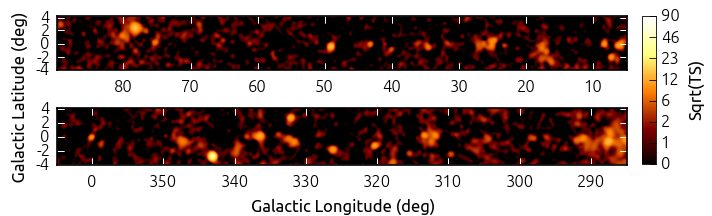# Flux and significance maps¶

## Introduction¶

The gammapy.estimators submodule includes low level functions to compute significance and test statistics images as well as some high level source detection method prototypes.

Detailed description of the methods can be found in [Stewart2009] and [LiMa1983].

Note that in Gammapy maps are stored as Numpy arrays, which implies that it’s very easy to use scikit-image or photutils or other packages that have advanced image analysis and source detection methods readily available.

## Computation of TS images¶Test statistics image computed using TSMapEstimator for an example Fermi dataset.

The gammapy.estimators module includes a high performance TSMapEstimator class to compute test statistics (TS) images for gamma-ray data. The implementation is based on the method described in [Stewart2009].

Assuming a certain source morphology, which can be defined by any astropy.convolution.Kernel2D instance, the amplitude of the morphology model is fitted at every pixel of the input data using a Poisson maximum likelihood procedure. As input data a counts, background and exposure images have to be provided. Based on the best fit flux amplitude, the change in TS, compared to the null hypothesis is computed using cash statistics.

To optimize the performance of the code, the fitting procedure is simplified by finding roots of the derivative of the fit statistics with respect to the flux amplitude. This approach is described in detail in Appendix A of [Stewart2009].

The following example shows how to compute a TS image for Fermi-LAT 3FHL galactic center data:

from gammapy.estimators import TSMapEstimator
from gammapy.datasets import MapDataset
from gammapy.maps import Map
from gammapy.irf import PSFKernel
from gammapy.modeling.models import BackgroundModel

counts =  Map.read("$GAMMAPY_DATA/fermi-3fhl-gc/fermi-3fhl-gc-counts-cube.fits.gz") background = Map.read("$GAMMAPY_DATA/fermi-3fhl-gc/fermi-3fhl-gc-background-cube.fits.gz")
exposure = Map.read("$GAMMAPY_DATA/fermi-3fhl-gc/fermi-3fhl-gc-exposure-cube.fits.gz") dataset = MapDataset( counts=counts, exposure=exposure, models=[BackgroundModel(background, datasets_names=["fermi-3fhl-gc"])], name="fermi-3fhl-gc" ) kernel = PSFKernel.read("$GAMMAPY_DATA/fermi-3fhl-gc/fermi-3fhl-gc-psf.fits.gz")

ts_estimator = TSMapEstimator()
result = ts_estimator.run(dataset, kernel.data)


The function returns an dictionary, that bundles all resulting maps. E.g. here’s how to find the largest TS value:

import numpy as np
np.nanmax(result['ts'].data)


## Computation of Li & Ma significance images¶

The method derived by [LiMa1983] is one of the standard methods to determine detection significances for gamma-ray sources. Using the same prepared Fermi dataset as above, the corresponding images can be computed using the LiMaMapEstimator class:

from gammapy.estimators import LiMaMapEstimator
from gammapy.datasets import MapDataset
from gammapy.maps import Map
from gammapy.modeling.models import BackgroundModel

counts =  Map.read("$GAMMAPY_DATA/fermi-3fhl-gc/fermi-3fhl-gc-counts-cube.fits.gz") background = Map.read("$GAMMAPY_DATA/fermi-3fhl-gc/fermi-3fhl-gc-background-cube.fits.gz")

dataset = MapDataset(
counts=counts,
exposure=exposure,
models=[BackgroundModel(background, datasets_names=["fermi-3fhl-gc"])],
name="fermi-3fhl-gc"
)

lima_estimator = LiMaMapEstimator("0.2 deg")
result = lima_estimator.run(dataset)


The function returns a dictionary, that bundles all resulting maps such as significance, flux and correlated counts and excess images.

## Using gammapy.detect¶

Tutorials that show examples using gammapy.detect: